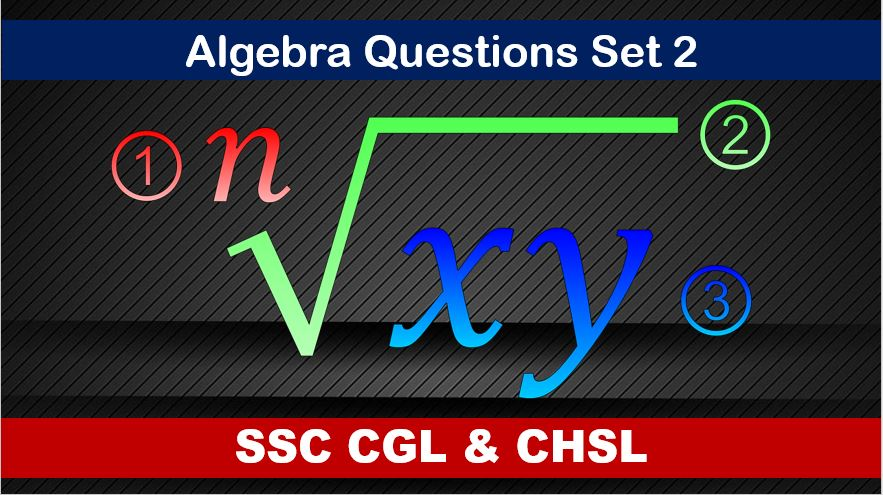Monday, September 27, 2021
Home > algebra > SSC CGL Algebra Questions and Solutions Set 2

# SSC CGL Algebra Questions and Solutions Set 2SSC CGL CHSL Algebra
Algebra Questions Sets
Set 1Set 2Set 3Set 4Set 5
Set 6

SSC CGL Algebra Questions and Solutions Set 2 : Ques No 1

If a = x + y, b = x – y and c = x + 2y, then the value of a2 + b2 + c2 is

Options:

A. 4y2

B. 5y2

C. 6y2

D. 7y2

Solution:

SSC CGL Algebra Questions and Solutions Set 2 : Ques No 2

If a + b = 5 and a2 + b2 = 13, then the value of (a – b) is (where a > b)

Options:

A. 1

B. -1

C. 2

D. -1

Solution:

SSC CGL Algebra Questions and Solutions Set 2 : Ques No 3

If x2 + x – 6 = 0 and x2 + 6x + 9 = 0, then the value of x is

Options:

A. 2

B. 3

C. -2

D. -3

Solution:

SSC CGL Algebra Questions and Solutions Set 2 : Ques No 4

If x = a + 1/a and y = a – 1/a, then the value of x4 + y4 – 2x2y2 is

Options:

A. 24

B. 18

C. 16

D. 12

Solution:

SSC CGL Algebra Questions and Solutions Set 2 : Ques No 5

If x + 9/x = 6, then the value of (x2 + 9/x2) is

Options:

A. 8

B. 9

C. 10

D. 12

Solution:

SSC CGL Algebra Questions and Solutions Set 2 : Ques No 6

If x1/3 + y1/3 = z1/3, then the value of (x + y + z) + 27xyz is

Options:

A. -1

B. 1

C. 0

D. 27

Solution:

SSC CGL Algebra Questions and Solutions Set 2 : Ques No 7

If (x + y – z)2 + (y + z – x)2 + (z + x – y)2 = 0, then the value of x + y + z is

Options:

A. √3

B. 3√3

C. 3

D. 0

Solution:

SSC CGL Algebra Questions and Solutions Set 2 : Ques No 8

If a ≠ b, then which of the following is true?

Options:

A. (a + b) / 2 = √ab

B. (a + b) / 2 < √ab

C. (a + b) / 2 > √ab

D. All of these

Solution:

SSC CGL Algebra Questions and Solutions Set 2 : Ques No 9

If a /b = c / d = e / f = 3, then the value of (2a2 + 3c2 + 4e2) / (2b2 + 3d2 + 4f2) is

Options:

A. 2

B. 3

C. 4

D. 9

Solution:

SSC CGL Algebra Questions and Solutions Set 2 : Ques No 10

If x + 1/x = 2, where x is real, then the value of x17 + 1/x19 is

Options:

A. 1

B. 0

C. 2

D. -2

Solution:

SSC CGL Algebra Questions and Solutions Set 2 : Ques No 11

The sum of A and B age is 43 years. 11 year hence, A‘s age will be 7/6 times B‘s age then. Find B‘s present age is

Options:

A. 22 years

B. 20 years

C. 24 years

D. 19 years

SSC CGL Algebra Questions and Solutions Set 2 : Ques No 12

A, B, C and D play a game of cards. A says to B “If I give you 8 cards, you will have as many as C has and I shall have 3 less than what C has. Also if I take 6 cards from C, I shall have twice as many as D has”. If B and D together have 50 cards, how many cards have A got?

Options:

A. 40

B. 37

C. 30

D. 27

SSC CGL Algebra Questions and Solutions Set 2 : Ques No 13

Ram’s age was square of number last year and it will be cube of a number next year. How long must he wait before his age is again a cube of a number?

Options:

A. 10 years

B. 38 years

C. 39 years

D. 46 years

SSC CGL CHSL Algebra
Algebra Questions Sets
Set 1Set 2Set 3Set 4Set 5
Set 6
Get Online Maths Tuition by AMBiPi for Class 8 to 12